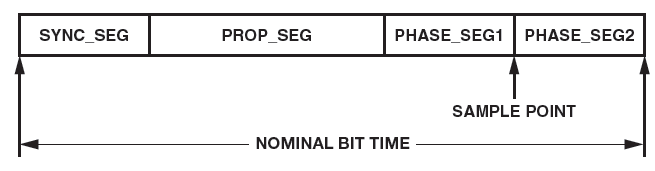# How to Calculate the Controller Parameters for an Isolated CAN (Controller Area Network) Network to Run at 1 Mbps

CAN is a differential signaling standard that is widely used in the automotive, industrial, and instrumentation industries. It is used for serial communication between systems that can be connected to different power systems, often over long distances. Due to these environments, galvanic isolation is often used in order to break ground loops or provide physical safety.

Isolated CAN networks will have an increased propagation delay over nonisolated CAN networks, and it can often be challenging to design. Figure 1 shows an example of an isolated CAN node using the ADM3053 signal and power isolated CAN transceiver for calculating the necessary CAN controller parameters to communicate at 1 Mbps over a 20 m cable.Figure 1. Isolated CAN node using the ADM3053 signal and power isolated CAN transceiver.

A CAN bit consists of four separate time segments, synchronization segment (SYNC_SEG), propagation segment (PROP_SEG), phase segment 1 (PHASE_SEG1), and phase segment 2 (PHASE_SEG2). These time segments are programmable in the CAN controller and are critical in calculating the setup parameters for the CAN controller. Figure 2 shows the different segments of a nominal bit time.Figure 2. CAN nominal bit time.

The following assumptions were made during the calculation:

• Cable length of 20 m
• Data rate or bit rate of 1 Mbps
• Cable propagation delay of 5 ns/m
• CAN controller oscillator frequency of 36 MHz

CAN uses bitwise arbitration, which allows different nodes to contend for access on the bus. This causes more than one node to be able to transmit data at a time. A transmitting node will have to sample the data on the bus in order to determine if it has won arbitration. Due to the propagation delay of the system, the controller has to compensate for when to sample each bit. Programming the PROP_SEG into the controller will allow this compensation and can be calculated as follows:

The propagation delay from TxD to RxD for the ADM3053 is 250 ns (max).

The physical delay of the cable is equal to 5 ns/m multiplied by a length of 20 m equaling a total of 100 ns.

This gives a total propagation time through the system and back as 2 (physical delay + transceiver prop delay) equaling 700 ns (2 × (100 + 250) = 700 ns).

In order to program the controller, the registers have to be setup as an integer multiple of a unit called a time quantum. The time period of the time quantum is equal to the CAN system clock, which in this case is 28 ns.

A time quantum of 28 ns will give 36 (1000/28 = 36) time quanta per bit.

PROP_SEG = ROUND_UP ( 700 ns/28 ns) = 25 Time Quanta.

From 36 time quanta per bit, subtract 25 for PROP_SEG and 1 for SYNC_SEG. This leaves 5 time quanta for PHASE_SEG1 and PHASE_SEG2.

Due to the tolerance in the CAN system clock, an accumulated phase error will occur. This requires the system to resynchronize through the resynchronization jump (RJW). This is determined as the smaller value of 4 and PHASE_SEG1.

This allows us to calculate the oscillator tolerance required for the system:

The smaller of these two values is the required oscillator tolerance, 0.5%.

This calculation gives the following setup parameters:

• SYNC_SEG = 1
• PROP_SEG = 25
• PHASE_SEG1 = 5
• PHASE_SEG2 = 5
• RJW = 4